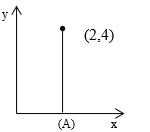Work done by Diff type of forces
Question

# A force  $\overline{\mathrm{F}}=\left({\mathrm{y}}^{2}-{\mathrm{x}}^{2}+{\mathrm{z}}^{2}\right)\stackrel{^}{\mathrm{i}}+\left(3\mathrm{xy}-5\mathrm{z}\right)\stackrel{^}{\mathrm{j}}+4\mathrm{z}\stackrel{^}{\mathrm{k}}$ is applied on a particle from the point (0,0,0) to the point (2,4,0) in the path shown . If  ${\mathrm{W}}_{\mathrm{A}}$  is the work done by the force in the paths A, then ${\mathrm{W}}_{\mathrm{A}}$ =?

Moderate
Solution

##The work done by the external force is $\int \stackrel{\to }{\mathrm{F}}.\stackrel{\to }{\mathrm{dS}}$ . Now here in this case the z coordinate of the motion of the particle is zero. $\stackrel{\to }{\mathrm{F}}=\left({\mathrm{y}}^{2}-{\mathrm{x}}^{2}\right)\stackrel{^}{\mathrm{i}}+3\mathrm{xy}\stackrel{^}{\mathrm{j}}$Hence ${\text{W}}_{\text{A}}={\int }_{\text{0}}^{\text{2}}-{\text{x}}^{\text{2}}\text{dx}+{\int }_{\text{0}}^{\text{4}}\text{3xy\hspace{0.17em}dy\hspace{0.17em}}={\left[\frac{-{\text{x}}^{\text{3}}}{\text{3}}\text{\hspace{0.17em}\hspace{0.17em}}\right]}_{0}^{2}\text{\hspace{0.17em}}+{\left[3{\mathrm{y}}^{2}\right]}_{0}^{4}=\frac{-8}{3}+48=\frac{136}{3}$

Get Instant Solutions